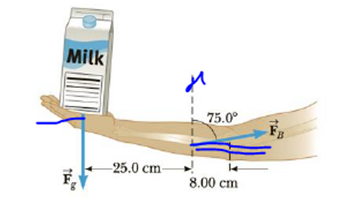Chapter 8, Problem 19P

Chapter
Section
Textbook Problem

A cook holds a 2.00-kg carton of milk at arm's length P8.19). What force F → B must be exerted by the biceps muscle? (Ignore the weight of the forearm.)Figure P8.19

To determine
The force exerted by the biceps muscle.

Explanation

Given info: The mass of the carton of milk is 2.00kg , the acceleration due to gravity is 9.80m/s2 , the length of the arm from the left side of the pivot point is 25.0cm , the length of the arm from right side of the pivot point is 8.00cm , the angle of the force by the biceps is 75.0° .

Explanation: At equilibrium condition, the sum of torques on her arm length is τ=0=(l1+l2)mgl2FBcosθ by ignoring the weight of the forearm.

The formula for the force exerted by the biceps muscle is,

FB=(l1+l2)mgl2cosθ

• l1 is length of the arm from the left side of the pivot point.
• l2 is length of the arm from right side of the pivot point.
• m is mass of the carton of milk.
• g is acceleration due to gravity.
• θ is angle of the force by the biceps

Still sussing out bartleby?

Check out a sample textbook solution.

See a sample solution

The Solution to Your Study Problems

Bartleby provides explanations to thousands of textbook problems written by our experts, many with advanced degrees!

Get Started